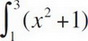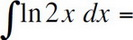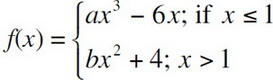# AP Calculus AB Practice Test 5

### Test Information7 questions21 minutes

Calculator Allowed

1. If the definite integralis approximated by using the Trapezoid Rule with n = 4, the error is

2. The radius of a sphere is increasing at a rate proportional to itself. If the radius is 4 initially, and the radius is 10 after two seconds, what will the radius be after three seconds?

3. Use differentials to approximate the change in the volume of a sphere when the radius is increased from 10 to 10.02 cm.

4.5. If the function f(x) is differentiable and, then a =

6. Two particles leave the origin at the same time and move along the y-axis with their respective positions determined by the functions y1 = cos 2t and y2 = 4sin t for 0 < t < 6. For how many values of t do the particles have the same acceleration?

7. Find the distance traveled (to three decimal places) in the first four seconds, for a particle whose velocity is given by v(t) = 7e-t2, where t stands for time.